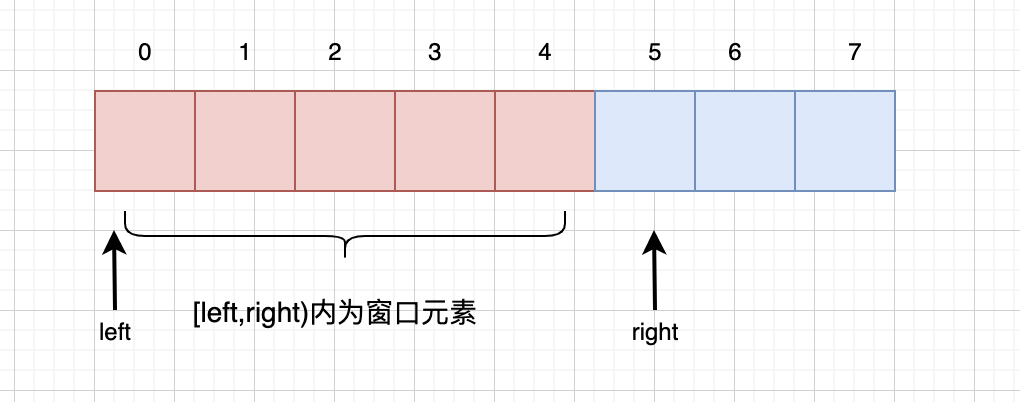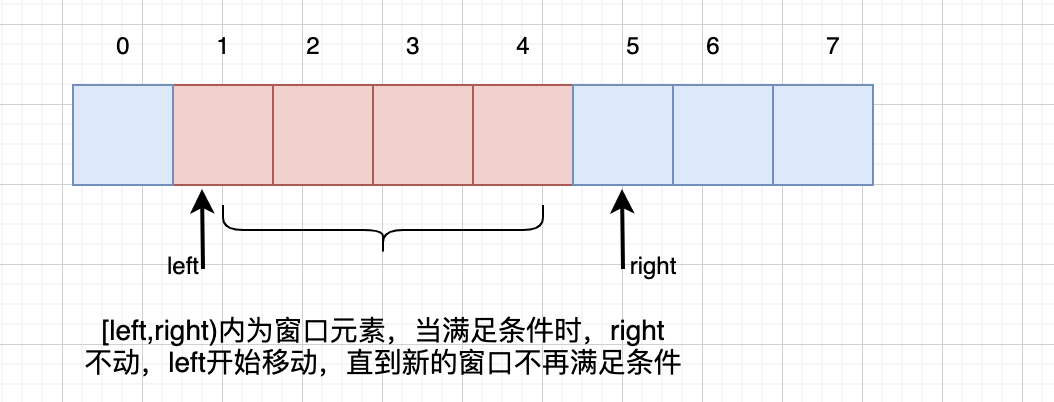墨滴2021/10/18  阅读：42  主题：默认主题

# 滑动窗口

## 基本概念

1. 一般给出的数据结构是数组或者字符串
2. 求取某个子串或者子序列最长最短等最值问题或者求某个目标值时
3. 该问题本身可以通过暴力求解

### 核心思路

1. 初始时，左右指针left,right都指向第0个元素，窗口为[left,right)，注意这里是左闭右开，因此初始窗口[0,0)区间没有元素，符合我们的初始定义
1. 开始循环遍历整个数组元素，判断当前right指针是否超过整个数组的长度，是退出循环，否则执行第3步

2. 然后right指针开始向右移动一个长度，并更新窗口内的区间数据3. 当窗口区间的数据满足我们的要求时，右指针right就保持不变，左指针left开始移动，直到移动到一个不再满足要求的区间时，left不再移动位置4. 执行第2步

``int left = 0,right =0;while(right指针未越界){  char ch = arr[right++];  //右指针移动，更新窗口  ...    //窗口数据满足条件 对于固定窗口而言，就是窗口的大小>=固定值；对于动态窗口，就是从left出发，窗口不断扩充，第一次满足题意的位置  while(窗口数据满足条件){   //记录或者更新全局数据   ...      //右指针不动，左指针开始移动一位   char tmp = arr[left++];      //左指针移动，窗口缩小，更新窗口数据   ...  }  //返回结果  ...}``

## 实战

### LC438. 找到字符串中所有字母异位词

``给定两个字符串 s 和 p，找到 s 中所有 p 的 异位词 的子串，返回这些子串的起始索引。不考虑答案输出的顺序。异位词 指由相同字母重排列形成的字符串（包括相同的字符串）。输入: s = "cbaebabacd", p = "abc"输出: [0,6]解释:起始索引等于 0 的子串是 "cba", 它是 "abc" 的异位词。起始索引等于 6 的子串是 "bac", 它是 "abc" 的异位词。``

#### 分析

``    public List<Integer> findAnagrams(String s, String p) {        List<Integer> res = new ArrayList<>();        Map<Character, Integer> map = new HashMap<>();        Map<Character, Integer> window = new HashMap<>();        char[] pattern = p.toCharArray();        char[] arr = s.toCharArray();        for (char a : pattern) {            map.put(a, map.getOrDefault(a, 0) + 1);        }        int left = 0, right = 0;        int valid = 0;        while (right < s.length()) {            char ch = arr[right];            right++;            /**             * 新元素加入窗口：什么时候当前元素可以加入窗口并新增valid计数?             * 当前元素在 map 中，那么把它加入到window中，加入后，如果在窗口内 该元素的数量和要求该元素数量一致，那么valid++,即该元素满足要求             */            if (map.containsKey(ch)) {                //加入窗口                window.put(ch, window.getOrDefault(ch, 0) + 1);                // 不能写== 比较的是两个地址                if (window.get(ch).equals(map.get(ch))) {                    valid++;                }            }            //判断窗口内元素是否满足条件,两个条件：1）固定窗口，当前窗口数量等于 模式字符串p的长度 2)当前窗口内组成的字符串和模式字符串 是异位词            while (right - left == p.length()) {                //固定窗口，判断当前窗口是否是异位词,如果是说明找到了一个位置，把left加入到结果集中                if (valid == map.size()) {                    res.add(left);                }                //right 不动，左指针开始向右，arr[left]元素移出窗口,同时该元素在window中对于的数目也要减去1                char tmp = arr[left];                left++;                if (map.containsKey(tmp)) {                    if (map.get(tmp).equals(window.get(tmp))) {                        valid--;                    }                    window.put(tmp, window.get(tmp) - 1);                }            }        }        return res;    }``

### LC76. 最小覆盖子串

#### 分析

``    public String minWindow(String s, String t) {        int start = 0, len = s.length() + 1;        char[] pattern = t.toCharArray();        char[] arr = s.toCharArray();        Map<Character, Integer> map = new HashMap<>();        Map<Character, Integer> window = new HashMap<>();        for (char a : pattern) {            map.put(a, map.getOrDefault(a, 0) + 1);        }        int left = 0, right = 0, valid = 0;        while (right < s.length()) {            char ch = arr[right++];            if (map.containsKey(ch)) {                window.put(ch, window.getOrDefault(ch, 0) + 1);                if (window.get(ch).equals(map.get(ch))) {                    valid++;                }            }            //满足条件的窗口 这里不是固定窗口，对窗口大小不限制，当map和window中的数据一致，满足条件，开始收缩            while (valid == map.size()) {                // 当前已经满足要求，更新并记录数据，同时收缩窗口                if (right - left < len) {                    start = left;                    len = right - left;                }                char tmp = arr[left++];                if (map.containsKey(tmp)) {             // 当前要移出的字符种类和数量和map一致，移出后不一致，valid--                    if (window.get(tmp).equals(map.get(tmp))) {                        valid--;                    }                    window.put(tmp, window.get(tmp) - 1);                }            }        }        return len == s.length()+1 ? "" : s.substring(start, start + len);    }``

## 最后2021/10/18  阅读：42  主题：默认主题